Maths
Magic tricks
Author
Marion Montocchio, Lycée Charles Baudelaire
Target Group
15-17 years old students
Brief Description
Magic tricks

Share

 MAGIC TRICKS

Mathematics is full of curiosities which can be used as the basis of seemingly « magic » tricks. Many “magicians” are also keen on mathematics : Lewis Carroll, for example, of Alice in Wonderland fame, was a mathematician who loved magic and puzzles.

Here are a few amazing predictions that require minimal equipment, simple computations or reasoning… an apprentice magician who will no doubt be amused and captivated.

The science of magic or the magic of science ?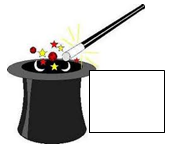Are predictions more fascinating

ð  when they seem magical ?

ð  or when we find a way to explain them, and then can marvel at their simplicity, originality, or beauty ?

 MAGIC TRICKS                              A TRICK WITH DICE

A player randomly piles up three dice, then announces the number  appearing face-up on the top dice.

The magician immediately guesses the sum of all numbers on the hidden horizontal faces (both top and bottom) of the three dice.

How ?

In fact, he merely has to calculate :    21-x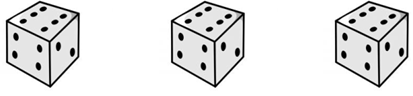Question

Explain why the trick works.

 MAGIC TRICKS                              A MAGIC TABLE

Consider the following table :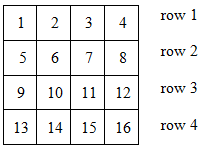In this table, have a volunteer select any number from row1.

Cross out all other numbers from the same column as the chosen number.

Then have the volunteer select any number from row 2, excluding the crossed-out column, and again cross out all numbers from its column.

Repeat for rows 3 and 4.

Then have the volunteer calculate the sum of the four selected numbers.

Your prediction ? 34, of course !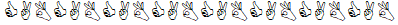Questions

1.    Why 34 ? Explain how this works.

2.    Can this trick be extended to a table with n rows and n columns, for any integer n ? What would be your prediction in this case ?MAGIC TRICKS                        A CARD  TRICK   :       Reading minds !

Take 27 cards from a deck of  32.

Have a player silently select a “favourite” card among the 27.

è Deal the cards (face up) into 3 piles of 9 cards each.

Then, the player indicates which pile contains the selected card.

Turn over and gather up the three piles, but quietly ensure that the designated pile is on the top.

è Repeat the previous step.

è Repeat the previous step again.

Finally, reveal your powers of mind-reading : not only did you guess the selected card, but you arranged for it to appear as the top card of the deck.

Questions

1.

Why does this card trick work ?

2.

This trick uses 27 cards, 3 piles, 3 steps.

Could a similar trick be performed with different numbers of cards, piles and steps ?

Predicting a sum

Why does the magic formula give the correct answer ?     S = 13(p4) + r

13 : the number of different cards in a color (diamonds for instance)

4 : the number of ace, the number of colors ( diamonds, hearts, spades, clubs)

the minimum number of piles

We can expand the formula :

13(p4) + r = 13p - 52+r

52 : the total number of cards in a full deck

_______________________________________________________________________________________________

Now we need to understand the formula.

First, let us take an example :

 Value of the first card +            left-over cards Number of cards we put on top of the first card

p=               (number of piles)

r=               (number of left-over cards)

S =        +        +       +       +       +        =                   (the sum of the first cards)

13(p-4)+r =

Then let us try to generalize :

 Value of the first card x1 x2 x3 xp +   r    left-over cards Number of cards we put on top of the first card 12-x1 12-x2 12-x3 12-xp

In pile p, there is :  12 - xp+ 1 = 13 - xp

There are __52___ cards in total in a full deck.

So we get :

++  …   ++    = 52

__p__ times

Û 13 x p - x1 - x2 - ... - xp + r = 52                                                                                                   ,

Û 13 x p - (x1 - x2 - ... - xp) + r = 52                                       because S = …………………………………………………

Û13 p - S + r = 52

ÛS = 13p -52 + r

ÛS = 13(p - 4) + r

Predicting a sum

Why does the magic formula give the correct answer ?     S = 13(p4) + r

13 :

4 :

We can expand the formula :

13(p4) + r =

52 :

_______________________________________________________________________________________________

Now we need to understand the formula.

First, let us take an example :

 Value of the first card +            left-over cards Number of cards we put on top of the first card

p=               (number of piles)

r=               (number of left-over cards)

S =        +        +       +       +       +        =                   (the sum of the first cards)

13(p-4)+r =

Then let us try to generalize :

 Value of the first card +            left-over cards Number of cards we put on top of the first card

In pile p, there is :

There are _____ cards in total in a full deck.

So we get :

+                         +  …   +                       +      =

____ times

Û

Û

Û                                                                                                              because S = …………………………………………………

Û

Û

 MAGIC TRICKS                          CARD  TRICK   :       Predicting a sum

The trick :

Ask someone in the audience to carry out the following task with a full deck of 52 cards while your back is turned so you cannot see.

è Shuffle  the cards.

è Place a card face down on the table, but note the value of the card ( here we count aces as 1, and jacks, queens, kings as 10 ; and the other cards as their own value).

è Place cards in a pile on top of the first card, counting from the value of the first card, up to 12. Thus, if a “7” is face down, there will be 5 more cards placed on top of it and this pile is now completed.

è Place another  card face down, noting the value, and repeat the procedure  in previous step.

è Repeat building piles until there are no more cards, or until it is not possible to complete a pile.

è Announce the number of left-over cards and the number of piles.

Then , you turn around, furrow your braw in a look of intense concentration for a few seconds, and announce to the audience the sum of the values of the cards on the bottom of the piles.

What is amazing (even to you ! ) is that you always get this right.

What do you do ?

In fact, there is a “magic formula” involved.

If p is the number of piles of cards and r the number of left-over cards, then the predicted sum is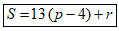Question  :        Why does the magic formula give the correct answer ?

Hint :      See how each pile is made.

-    If the bottom card has value n, find out how many cards the pile contains.

-    Now, what is the number of cards used for p piles with bottom values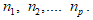Write down this number in terms of S.

-    What equation can we get ?

-     Solve this equation to find the result : S is ……

 MAGIC TRICKS                              A TRICK WITH DICEYou just need to know that the sum of the two numbers on each pair of opposite sides of a die is 7.

(indeed 6+1  5+2 or 3+4)

So if the top number is , then the sum to be guessed is :  21 - x

 MAGIC TRICKS                              A MAGIC TABLE

CABCABCABCABCABCABCABCAB

Row 1 : 0 + 1/2/3/4

Row 2 : 4 + 1/2/3/4

Row 3 : 8 + 1/2/3/4

Row 4 : 12 + 1/2/3/4

Total =0+4+8+12+  1+2+3+4 = 34 !

Column 1 : 4j+1                                 j?E={0,1,2,3}

Column 2 : 4k+2

Column 3 : 4l+3

Column 4 : 4m+4

Total=4(j+k+l+m)+1+2+3+4=34

Can be extended to an n-table : then the sum to predict will be  n(n²+1)/2

 MAGIC TRICKS                        A CARD  TRICK   :       Reading minds !

Let S be the selected card.

After the 1st distribution, the designated pile with S is gathered with the two others but is on top – thus S is among the 9 first cards to be displayed on the next deal.

After the 2nd round, S is among the 3 first cards to be displayed on the next deal.

And and the 3rd round, S is necessarily the top card !

A similar trick could be performed with for example 4^4 cards, 4 piles and 4 steps provided that you could find 256 cards ans an audience with enough patience !

 MAGIC TRICKS                          CARD  TRICK   :       Predicting a sum

See how each pile is made : if the bottom card has value n, then the pile contains (12 – n ) + 1 cards.

( because we have the bottom card and 12 – n  cards over it). So, the pile contains 13– n cards.

Now, p piles with bottom values respectively n1, n2, ......np use (13– n1)+ (13– n2)+.......+ (13– np) cards altogether ; which is 13 p – (n1+ n2+....+ np) or    13 p – S  where S is the sum to predict.

Since there are r cards left over and 52 cards in total, we get the following equation :

13 p – S + r = 52 .

It’s easy to find out S : S = 13p – 52 + r

so that,  S = 13 ( p – 4) +r

## Related files

 00 magic tricks 129 KB 01_trick with dice 145 KB 02_magic table 30 KB 03_27 cards_reading minds 138 KB 04 Predicting a sum _ solution 469 KB 04 Predicting a sum _ student help 458 KB 04_52 cards_predicting a sum 143 KB 05_answers 1_2_3_4 353 KB
If you have tried out a lesson, please fill the next form to help us to improve it: